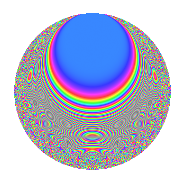Properties

 Label 2006.2.aLevel 2006 Weight 2 Character orbit a Rep. character $$\chi_{2006}(1,\cdot)$$ Character field $$\Q$$ Dimension 79 Newforms 23 Sturm bound 540 Trace bound 5

Related objects

Defining parameters

 Level: $$N$$ = $$2006 = 2 \cdot 17 \cdot 59$$ Weight: $$k$$ = $$2$$ Character orbit: $$[\chi]$$ = 2006.a (trivial) Character field: $$\Q$$ Newforms: $$23$$ Sturm bound: $$540$$ Trace bound: $$5$$ Distinguishing $$T_p$$: $$3$$, $$5$$, $$11$$, $$31$$

Dimensions

The following table gives the dimensions of various subspaces of $$M_{2}(\Gamma_0(2006))$$.

Total New Old
Modular forms 274 79 195
Cusp forms 267 79 188
Eisenstein series 7 0 7

The following table gives the dimensions of the cuspidal new subspaces with specified eigenvalues for the Atkin-Lehner operators and the Fricke involution.

$$2$$$$17$$$$59$$FrickeDim.
$$+$$$$+$$$$+$$$$+$$$$7$$
$$+$$$$+$$$$-$$$$-$$$$12$$
$$+$$$$-$$$$+$$$$-$$$$13$$
$$+$$$$-$$$$-$$$$+$$$$8$$
$$-$$$$+$$$$+$$$$-$$$$13$$
$$-$$$$+$$$$-$$$$+$$$$6$$
$$-$$$$-$$$$+$$$$+$$$$7$$
$$-$$$$-$$$$-$$$$-$$$$13$$
Plus space$$+$$$$28$$
Minus space$$-$$$$51$$

Trace form

 $$79q$$ $$\mathstrut -\mathstrut q^{2}$$ $$\mathstrut +\mathstrut 79q^{4}$$ $$\mathstrut -\mathstrut 2q^{5}$$ $$\mathstrut +\mathstrut 4q^{6}$$ $$\mathstrut +\mathstrut 8q^{7}$$ $$\mathstrut -\mathstrut q^{8}$$ $$\mathstrut +\mathstrut 83q^{9}$$ $$\mathstrut +\mathstrut O(q^{10})$$ $$79q$$ $$\mathstrut -\mathstrut q^{2}$$ $$\mathstrut +\mathstrut 79q^{4}$$ $$\mathstrut -\mathstrut 2q^{5}$$ $$\mathstrut +\mathstrut 4q^{6}$$ $$\mathstrut +\mathstrut 8q^{7}$$ $$\mathstrut -\mathstrut q^{8}$$ $$\mathstrut +\mathstrut 83q^{9}$$ $$\mathstrut -\mathstrut 6q^{10}$$ $$\mathstrut -\mathstrut 12q^{11}$$ $$\mathstrut +\mathstrut 10q^{13}$$ $$\mathstrut -\mathstrut 16q^{15}$$ $$\mathstrut +\mathstrut 79q^{16}$$ $$\mathstrut +\mathstrut 3q^{17}$$ $$\mathstrut -\mathstrut 5q^{18}$$ $$\mathstrut +\mathstrut 20q^{19}$$ $$\mathstrut -\mathstrut 2q^{20}$$ $$\mathstrut +\mathstrut 16q^{21}$$ $$\mathstrut -\mathstrut 8q^{22}$$ $$\mathstrut -\mathstrut 16q^{23}$$ $$\mathstrut +\mathstrut 4q^{24}$$ $$\mathstrut +\mathstrut 73q^{25}$$ $$\mathstrut +\mathstrut 2q^{26}$$ $$\mathstrut +\mathstrut 8q^{28}$$ $$\mathstrut +\mathstrut 14q^{29}$$ $$\mathstrut +\mathstrut 8q^{30}$$ $$\mathstrut +\mathstrut 8q^{31}$$ $$\mathstrut -\mathstrut q^{32}$$ $$\mathstrut +\mathstrut 16q^{33}$$ $$\mathstrut -\mathstrut q^{34}$$ $$\mathstrut -\mathstrut 24q^{35}$$ $$\mathstrut +\mathstrut 83q^{36}$$ $$\mathstrut +\mathstrut 18q^{37}$$ $$\mathstrut +\mathstrut 12q^{38}$$ $$\mathstrut +\mathstrut 8q^{39}$$ $$\mathstrut -\mathstrut 6q^{40}$$ $$\mathstrut +\mathstrut 14q^{41}$$ $$\mathstrut +\mathstrut 8q^{42}$$ $$\mathstrut +\mathstrut 4q^{43}$$ $$\mathstrut -\mathstrut 12q^{44}$$ $$\mathstrut -\mathstrut 2q^{45}$$ $$\mathstrut +\mathstrut 8q^{46}$$ $$\mathstrut +\mathstrut 32q^{47}$$ $$\mathstrut +\mathstrut 95q^{49}$$ $$\mathstrut +\mathstrut 17q^{50}$$ $$\mathstrut +\mathstrut 10q^{52}$$ $$\mathstrut -\mathstrut 38q^{53}$$ $$\mathstrut +\mathstrut 16q^{54}$$ $$\mathstrut -\mathstrut 16q^{55}$$ $$\mathstrut +\mathstrut 8q^{57}$$ $$\mathstrut -\mathstrut 6q^{58}$$ $$\mathstrut -\mathstrut q^{59}$$ $$\mathstrut -\mathstrut 16q^{60}$$ $$\mathstrut +\mathstrut 42q^{61}$$ $$\mathstrut -\mathstrut 16q^{63}$$ $$\mathstrut +\mathstrut 79q^{64}$$ $$\mathstrut -\mathstrut 36q^{65}$$ $$\mathstrut +\mathstrut 40q^{66}$$ $$\mathstrut -\mathstrut 4q^{67}$$ $$\mathstrut +\mathstrut 3q^{68}$$ $$\mathstrut -\mathstrut 40q^{69}$$ $$\mathstrut -\mathstrut 24q^{70}$$ $$\mathstrut -\mathstrut 8q^{71}$$ $$\mathstrut -\mathstrut 5q^{72}$$ $$\mathstrut -\mathstrut 18q^{73}$$ $$\mathstrut -\mathstrut 18q^{74}$$ $$\mathstrut +\mathstrut 8q^{75}$$ $$\mathstrut +\mathstrut 20q^{76}$$ $$\mathstrut +\mathstrut 32q^{77}$$ $$\mathstrut +\mathstrut 48q^{78}$$ $$\mathstrut -\mathstrut 16q^{79}$$ $$\mathstrut -\mathstrut 2q^{80}$$ $$\mathstrut +\mathstrut 111q^{81}$$ $$\mathstrut +\mathstrut 6q^{82}$$ $$\mathstrut +\mathstrut 4q^{83}$$ $$\mathstrut +\mathstrut 16q^{84}$$ $$\mathstrut +\mathstrut 6q^{85}$$ $$\mathstrut -\mathstrut 20q^{86}$$ $$\mathstrut +\mathstrut 16q^{87}$$ $$\mathstrut -\mathstrut 8q^{88}$$ $$\mathstrut -\mathstrut 66q^{89}$$ $$\mathstrut -\mathstrut 6q^{90}$$ $$\mathstrut +\mathstrut 32q^{91}$$ $$\mathstrut -\mathstrut 16q^{92}$$ $$\mathstrut +\mathstrut 56q^{93}$$ $$\mathstrut +\mathstrut 48q^{95}$$ $$\mathstrut +\mathstrut 4q^{96}$$ $$\mathstrut -\mathstrut 10q^{97}$$ $$\mathstrut -\mathstrut 17q^{98}$$ $$\mathstrut -\mathstrut 28q^{99}$$ $$\mathstrut +\mathstrut O(q^{100})$$

Decomposition of $$S_{2}^{\mathrm{new}}(\Gamma_0(2006))$$ into irreducible Hecke orbits

Label Dim. $$A$$ Field CM Traces A-L signs $q$-expansion
$$a_2$$ $$a_3$$ $$a_5$$ $$a_7$$ 2 17 59
2006.2.a.a $$1$$ $$16.018$$ $$\Q$$ None $$-1$$ $$-1$$ $$-1$$ $$-5$$ $$+$$ $$-$$ $$+$$ $$q-q^{2}-q^{3}+q^{4}-q^{5}+q^{6}-5q^{7}+\cdots$$
2006.2.a.b $$1$$ $$16.018$$ $$\Q$$ None $$-1$$ $$-1$$ $$1$$ $$-1$$ $$+$$ $$+$$ $$+$$ $$q-q^{2}-q^{3}+q^{4}+q^{5}+q^{6}-q^{7}+\cdots$$
2006.2.a.c $$1$$ $$16.018$$ $$\Q$$ None $$-1$$ $$-1$$ $$2$$ $$4$$ $$+$$ $$-$$ $$+$$ $$q-q^{2}-q^{3}+q^{4}+2q^{5}+q^{6}+4q^{7}+\cdots$$
2006.2.a.d $$1$$ $$16.018$$ $$\Q$$ None $$-1$$ $$0$$ $$0$$ $$2$$ $$+$$ $$+$$ $$+$$ $$q-q^{2}+q^{4}+2q^{7}-q^{8}-3q^{9}+4q^{11}+\cdots$$
2006.2.a.e $$1$$ $$16.018$$ $$\Q$$ None $$-1$$ $$1$$ $$-3$$ $$-1$$ $$+$$ $$-$$ $$+$$ $$q-q^{2}+q^{3}+q^{4}-3q^{5}-q^{6}-q^{7}+\cdots$$
2006.2.a.f $$1$$ $$16.018$$ $$\Q$$ None $$-1$$ $$3$$ $$2$$ $$4$$ $$+$$ $$-$$ $$+$$ $$q-q^{2}+3q^{3}+q^{4}+2q^{5}-3q^{6}+4q^{7}+\cdots$$
2006.2.a.g $$1$$ $$16.018$$ $$\Q$$ None $$1$$ $$-3$$ $$-3$$ $$-1$$ $$-$$ $$-$$ $$+$$ $$q+q^{2}-3q^{3}+q^{4}-3q^{5}-3q^{6}-q^{7}+\cdots$$
2006.2.a.h $$1$$ $$16.018$$ $$\Q$$ None $$1$$ $$-1$$ $$-3$$ $$1$$ $$-$$ $$-$$ $$+$$ $$q+q^{2}-q^{3}+q^{4}-3q^{5}-q^{6}+q^{7}+\cdots$$
2006.2.a.i $$1$$ $$16.018$$ $$\Q$$ None $$1$$ $$-1$$ $$1$$ $$-1$$ $$-$$ $$+$$ $$+$$ $$q+q^{2}-q^{3}+q^{4}+q^{5}-q^{6}-q^{7}+\cdots$$
2006.2.a.j $$1$$ $$16.018$$ $$\Q$$ None $$1$$ $$0$$ $$0$$ $$2$$ $$-$$ $$-$$ $$+$$ $$q+q^{2}+q^{4}+2q^{7}+q^{8}-3q^{9}-6q^{11}+\cdots$$
2006.2.a.k $$1$$ $$16.018$$ $$\Q$$ None $$1$$ $$2$$ $$-2$$ $$2$$ $$-$$ $$+$$ $$+$$ $$q+q^{2}+2q^{3}+q^{4}-2q^{5}+2q^{6}+2q^{7}+\cdots$$
2006.2.a.l $$2$$ $$16.018$$ $$\Q(\sqrt{5})$$ None $$-2$$ $$0$$ $$0$$ $$-6$$ $$+$$ $$+$$ $$+$$ $$q-q^{2}-\beta q^{3}+q^{4}-\beta q^{5}+\beta q^{6}-3q^{7}+\cdots$$
2006.2.a.m $$2$$ $$16.018$$ $$\Q(\sqrt{5})$$ None $$2$$ $$1$$ $$-5$$ $$2$$ $$-$$ $$+$$ $$-$$ $$q+q^{2}+\beta q^{3}+q^{4}+(-2-\beta )q^{5}+\beta q^{6}+\cdots$$
2006.2.a.n $$3$$ $$16.018$$ 3.3.229.1 None $$-3$$ $$2$$ $$-2$$ $$3$$ $$+$$ $$+$$ $$+$$ $$q-q^{2}+(1+\beta _{2})q^{3}+q^{4}+(-1-\beta _{2})q^{5}+\cdots$$
2006.2.a.o $$3$$ $$16.018$$ 3.3.568.1 None $$3$$ $$-1$$ $$5$$ $$7$$ $$-$$ $$+$$ $$+$$ $$q+q^{2}-\beta _{1}q^{3}+q^{4}+(2-\beta _{1})q^{5}-\beta _{1}q^{6}+\cdots$$
2006.2.a.p $$4$$ $$16.018$$ 4.4.2225.1 None $$-4$$ $$-1$$ $$-5$$ $$-2$$ $$+$$ $$-$$ $$-$$ $$q-q^{2}-\beta _{1}q^{3}+q^{4}+(-1-\beta _{1}+\beta _{3})q^{5}+\cdots$$
2006.2.a.q $$4$$ $$16.018$$ $$\Q(\zeta_{20})^+$$ None $$-4$$ $$0$$ $$2$$ $$2$$ $$+$$ $$-$$ $$-$$ $$q-q^{2}+(-\beta _{2}+\beta _{3})q^{3}+q^{4}+(1+\beta _{1}+\cdots)q^{5}+\cdots$$
2006.2.a.r $$4$$ $$16.018$$ $$\Q(\sqrt{2}, \sqrt{5})$$ None $$4$$ $$-4$$ $$4$$ $$-4$$ $$-$$ $$+$$ $$-$$ $$q+q^{2}+(-1+\beta _{2})q^{3}+q^{4}+(1+\beta _{1}+\cdots)q^{5}+\cdots$$
2006.2.a.s $$4$$ $$16.018$$ 4.4.13676.1 None $$4$$ $$-3$$ $$3$$ $$-12$$ $$-$$ $$-$$ $$+$$ $$q+q^{2}+(-1+\beta _{3})q^{3}+q^{4}+(1-\beta _{3})q^{5}+\cdots$$
2006.2.a.t $$8$$ $$16.018$$ $$\mathbb{Q}[x]/(x^{8} - \cdots)$$ None $$8$$ $$5$$ $$-5$$ $$0$$ $$-$$ $$+$$ $$+$$ $$q+q^{2}+(1-\beta _{2})q^{3}+q^{4}+(-1+\beta _{7})q^{5}+\cdots$$
2006.2.a.u $$9$$ $$16.018$$ $$\mathbb{Q}[x]/(x^{9} - \cdots)$$ None $$-9$$ $$-1$$ $$7$$ $$0$$ $$+$$ $$-$$ $$+$$ $$q-q^{2}-\beta _{1}q^{3}+q^{4}+(1-\beta _{6})q^{5}+\beta _{1}q^{6}+\cdots$$
2006.2.a.v $$12$$ $$16.018$$ $$\mathbb{Q}[x]/(x^{12} - \cdots)$$ None $$-12$$ $$-3$$ $$-1$$ $$4$$ $$+$$ $$+$$ $$-$$ $$q-q^{2}-\beta _{1}q^{3}+q^{4}+\beta _{8}q^{5}+\beta _{1}q^{6}+\cdots$$
2006.2.a.w $$13$$ $$16.018$$ $$\mathbb{Q}[x]/(x^{13} - \cdots)$$ None $$13$$ $$7$$ $$1$$ $$8$$ $$-$$ $$-$$ $$-$$ $$q+q^{2}+(1-\beta _{1})q^{3}+q^{4}+\beta _{8}q^{5}+(1+\cdots)q^{6}+\cdots$$

Decomposition of $$S_{2}^{\mathrm{old}}(\Gamma_0(2006))$$ into lower level spaces

$$S_{2}^{\mathrm{old}}(\Gamma_0(2006)) \cong$$ $$S_{2}^{\mathrm{new}}(\Gamma_0(17))$$$$^{\oplus 4}$$$$\oplus$$$$S_{2}^{\mathrm{new}}(\Gamma_0(34))$$$$^{\oplus 2}$$$$\oplus$$$$S_{2}^{\mathrm{new}}(\Gamma_0(59))$$$$^{\oplus 4}$$$$\oplus$$$$S_{2}^{\mathrm{new}}(\Gamma_0(118))$$$$^{\oplus 2}$$$$\oplus$$$$S_{2}^{\mathrm{new}}(\Gamma_0(1003))$$$$^{\oplus 2}$$